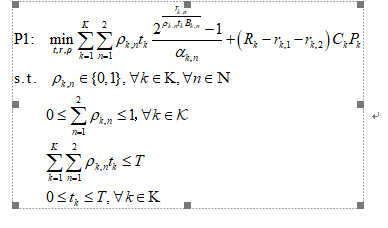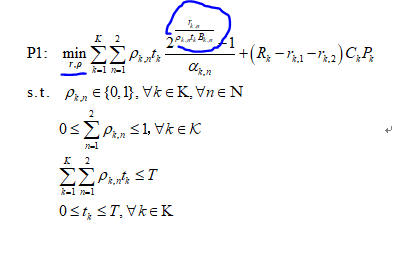# Wish List: Allow Limited DCP Non-Compliance as Front End for Non-Convex Solvers

could you tell me the reason for infeasible? I think it’s a really simple model,right?

could you tell me the reason for infeasible? I think it’s a really simple model,right?

You know the problem you are trying to solve. You build the model. You build the CVX program.

I wonder how come I should be in a better position than you to figure what is wrong with your model.
Sorry, but you have some hard work that only you can do.

Thanks，because I just don’t know the working principle of cvx very well, I’m not sure that its solving speed and difficulty have a greater relationship with which parameter in my model. Before, I thought it was the size of R that affected the results of the solution. Now it seems It may also be K, that is, no matter how big T and how small R I give it, as long as the value of K becomes larger, the solution speed of cvx will slow down, and even lead to unsolvable problems. I can’t be sure whether my thinking is correct.

Hi, Mark, so rorry to disturb you again.
My optimization model is as follows.Actually, the variable t is average-distribution by all user k, i.e. t=T/K for all k, so the variable is only binary rho and continous variable r, which means the transmission data volume. Could you tell me how to express the objective function of the following problem in cvx that can make it looks like convex?First you will have to linearize the products of binary and continuous, per section 2.8 of https://www.fico.com/en/resource-access/download/3217 .

Then, using the new product variable, say z_{k,n}, you can handle the first term inside the sums using the exponential cone construct.

y > 0; y*exp(x/y) <= z
which is entered into CVX as
{x,y,z} == exponential(1)

You will need to change from exponent base 2 to exponent base e, and take the rest of the stuff and put it in this standard form. I suggest you get rid of all the indices and sums to begin with, and get things correct in that simple case. Then modify to handle the extra complexity of indices. I leave you to work out the details - that way you will learn something.

And please don’t keep posting new questions in this same off-topic thread.

Thanks very much!But in the objective function of my problem, there is no product of continuous variable and binary variable ,the binary variable rho is divided by the continous variable r.So I’m not sure what z should be?

Sorry,I don’t know where to reply you in any other topic.r is not listed as a variable.

I am referring to \rho_{n,k}t_k

Perhaps I understand the problem you copied out of a book or paper better than you do?

I’m sorry, maybe I didn’t make it clear. In my question, there are three original optimization variables：r,t,rho. But now, as a comparison scheme of my original algorithm, I have evenly divided the total time of the system, that is, t=T/K for all k. So there are only two variables left at this time:r and rho.
I don’t know if I’m saying this to make you more clear about my problem?
Thanks!

You posted an image of a problem which lists the optimization variables. Now you say those aren’t your optimization variables - apparently you’ve modified the problem from the image you posted. I don’t see a product of r with any (other) variable. I don’t know what your problem is at this point.

I gave you the approach for entering the problem in the image into CVX. If you have now simplified the problem, perhaps it’s even easier to enter in CVX.I’m so sorry.
Now, there are only two variables in my problem,does this image make you more clear?

You don’t need to do any linearization. Just follow the exponential cone suggestion in my earlier post.

Actually, I’m not sure what z should mean in my problem. Does x mean rho and y mean r?

z is the new variable to replace the expression. i.e., replace y*exp(x/y) with z. I left it for you to figure out what x and y need to be to match the required form. I could do it, but I’m letting you do it. So please put in the effort. If you search on this forum, you can see some examples coded explicitly.

I got it,maybe y is rho,and x is r in my model.So i should write this expression{x,y,z} == exponential(1) as a cosntraint in cvx，right?
I’ll have a try tomorrow.Really thanks,goodnight.